7. Trigonometrical Functions

The common trig. functions are defined relative to a right-angled triangle.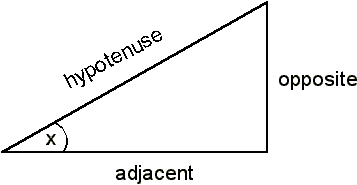• tan x = opposite/adjacent O/A

• sin x = opposite/hypotenuse O/H

• cos x = adjacent/hypotenuse A/H

This can be remembered using sOHcAHtOA (sin = OH, cos = AH, tan = OA)

In calculus, we need the angles measured in radians rather than degrees, with

2π radians in a circle = 360°

so that 1 radian = 360° / 2π.

The common points used:

For graphs of the common trig functions, see handout 2.

Inverse Trig. Functions

If y = sin x, then x = sin-1y (also called arcsin y)

y = cos x, then x = cos-1y (also called arccos y)

y = tan x, then x = tan-1y (also called arctan y).

Examples

1. sin x = 0.32, x = sin-10.32 = 18.7 = 0.33 rads

2. tan x = 0.87, x = tan-10.87 = 41 = 0.71 rads

Differentials of Trig functions

It can be shown that if

• y = sin x,= cos x

• y = cos x,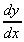= - sin x

• y = tan x,=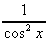We often meet compound functions in many applications and we can use the Product, Chain and Sequential rules as before.

Examples

1. y = sin(kx)= cos(kx).k = kcos (kx)         important

2. y = 3cos x - 4sin(x2)= -3sin x - 4 cos(x2).2x

= -3sin x - 8x.cos(x2)

3. y = 7sin(5x2) + 6ln {tan(5x)}= 7cos(5x2).10x +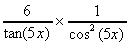5

= 70x.cos(5x2) +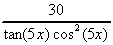4. Ψ(x) = Asin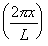, a typical wave function for an electron in an orbital.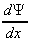= Acos.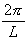=.cos.

8. Integration - Calculating Areas

In science, we often need to work out the area under a graph. For example the work done to move a charged particle a distance r through a potential difference V is given by the area under the potential vs distance curve.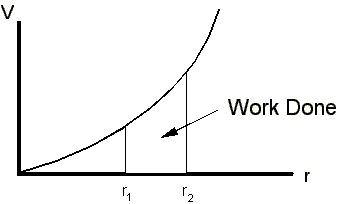Another example is the total distance travelled by an object moving at velocity v(t), (which is a function of time so that the object is accelerating), is given by the area under the v(t) vs t graph.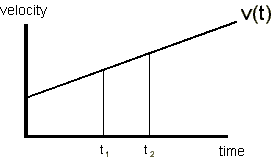Calculating Areas - counting squares

The most obvious way to calculate the area under a graph is to draw it on graph paper and count the squares.

Example What is the area under the curve y(x) = 2x2 from x = 1 to x = 3?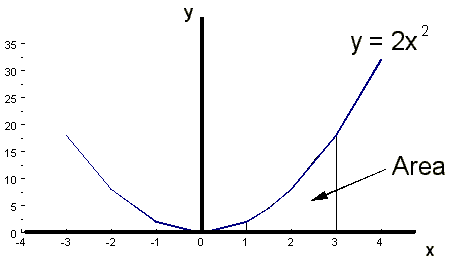a) Divide the area up into 2 trapezia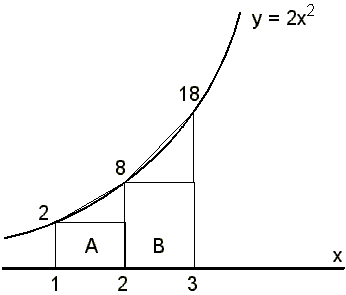Area of trapezium = Area of rectangle + area of triangle

= (length x width) + (½ x length x height)

Area of A = (1 x 2) + (½ x 1 x 6) = 5 units

Area of B = (1 x 8) + (½ x 1 x 10) = 13 units

Total Area = A+B = 5+13 = 18 sq. units

b) Now do it again with 4 trapeziaArea of A = (½ x 2) + (½ x ½ x 2½) = 15/8

Area of B = (½ x 4½) + (½ x ½ x 3½) = 33/8

Area of C = (½ x 8) + (½ x ½ x 4½) = 51/8

Area of D = (½ x 12½) + (½ x ½ x 5½) = 75/8

Total Area = A+B+C+D = 17.75 sq.units

You can see that as we divide up the area into smaller and smaller strips, the approximation to the area gets better and better. This is the basis for numerical solutions of areas (e.g. Simpson's Rule - see textbooks).

The actual value of the area will be achieved when we have an infinite number of strips, of width zero!

Needless to say we do not have to do this - there's a short cut - analytic integration

Integration - Notation

The area under a curve, y(x), which has been divided up into many strips of width x between the limits of x = a and x = b is given by adding up all the areas of the strips (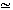width x height).

i.e. Area =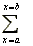(height of strip) x (width of strip)

Area =y(x). δx

Now, as x approaches 0 (i.e. the strips get vanishingly thin) we can replace the Σ with an integration sign which is an extended S, for sum.

So we get:The dx now serves to tell us what the name of the variable is - we say 'with respect to x'

This process is called integration

Integration as the Reverse of Differentiation

We know that the differential of y(x) = x3 is 3x2. The process of reversing this, whereby we generate a function from its derivative is integration.

i.e.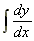= y(x), so that integration of a differential gives the original function.

and[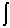y(x).dx] = y(x), so that differential of an integral gives the original function.

So, integration is the reverse process to differentiation

Compare other reversible functions such as:

• x2 and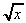• sin x and sin-1x

• ln x and ex.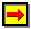Next lecture